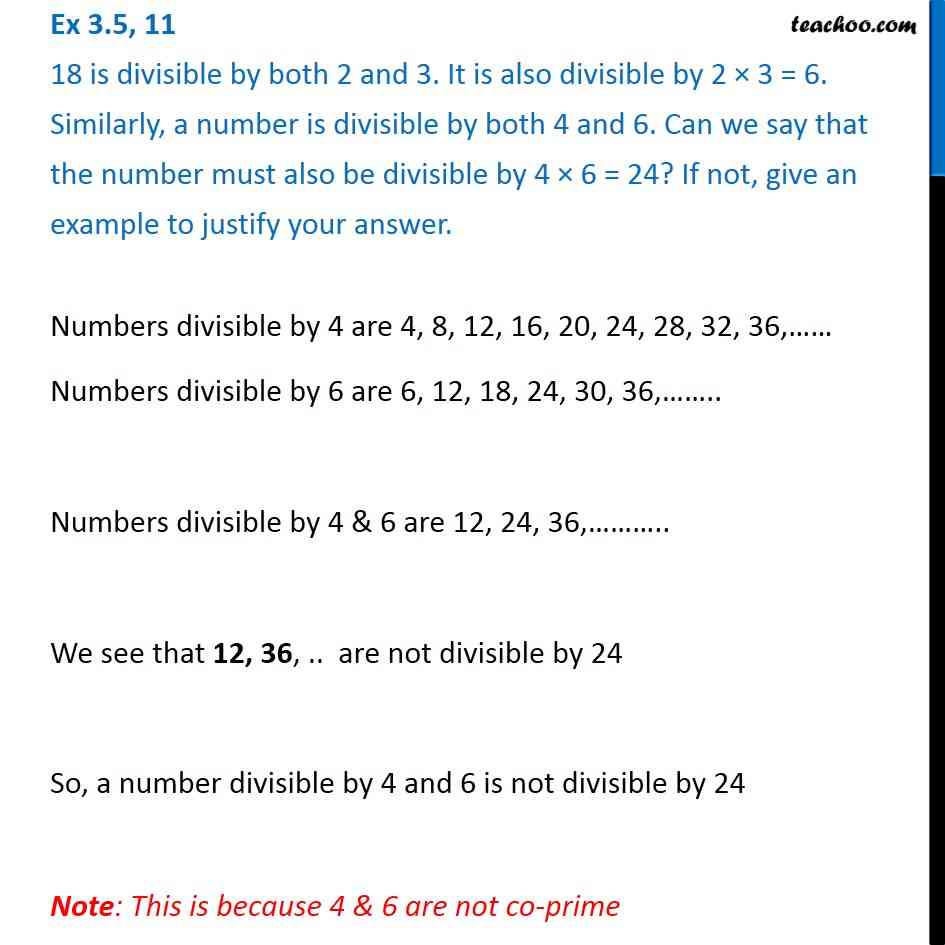Ex 3.5

Chapter 3 Class 6 Playing with Numbers
Serial order wiseGet live Maths 1-on-1 Classs - Class 6 to 12

### Transcript

Ex 3.5, 11 18 is divisible by both 2 and 3. It is also divisible by 2 × 3 = 6. Similarly, a number is divisible by both 4 and 6. Can we say that the number must also be divisible by 4 × 6 = 24? If not, give an example to justify your answer.Numbers divisible by 4 are 4, 8, 12, 16, 20, 24, 28, 32, 36,…… Numbers divisible by 6 are 6, 12, 18, 24, 30, 36,…….. Numbers divisible by 4 & 6 are 12, 24, 36,……….. We see that 12, 36, .. are not divisible by 24 So, a number divisible by 4 and 6 is not divisible by 24 Note: This is because 4 & 6 are not co-prime